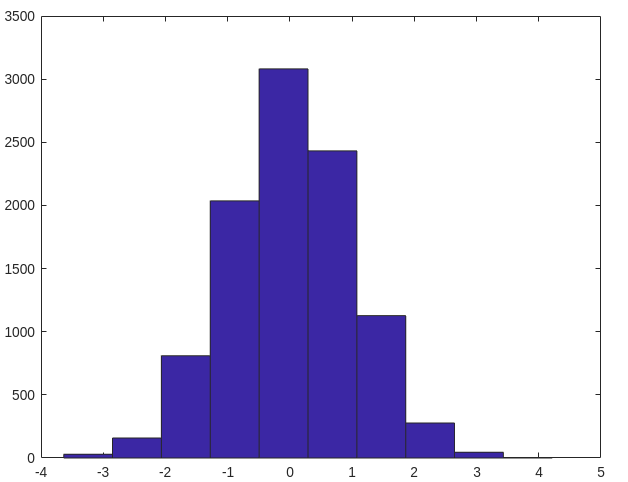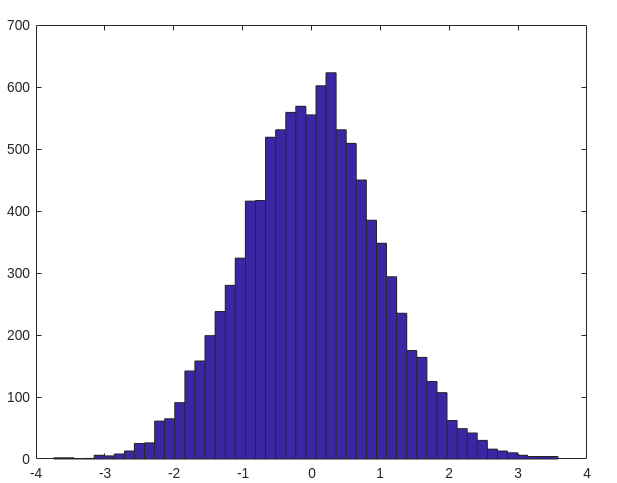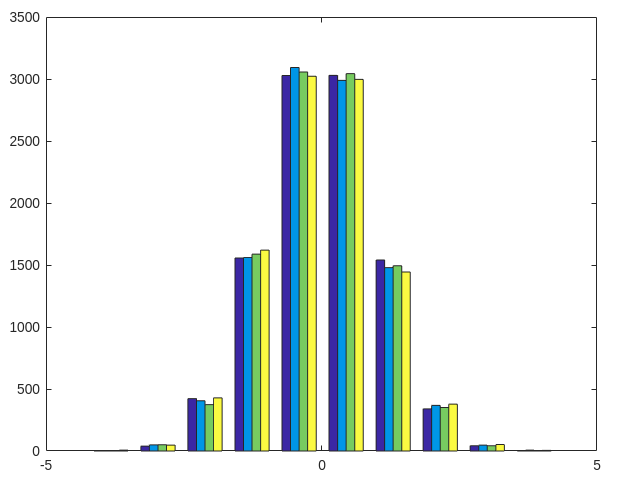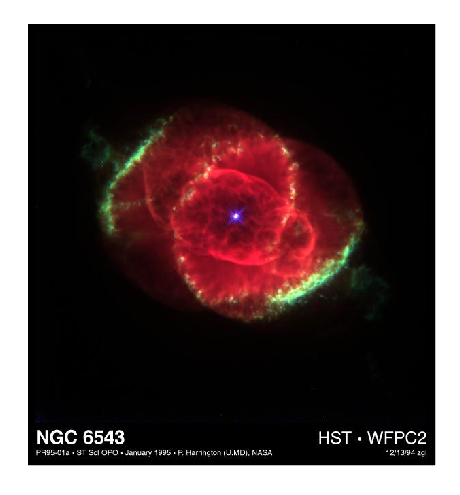# How to plot a Histogram in MATLAB ?

A Histogram is a diagrammatic representation of a group of data over user-specified ranges. Basically, the histogram contains several bins. Bins are non-overlapping intervals in which the data is spread. In MATLAB we have a function named hist() which allows us to plot a bar graph.

Syntax:

```hist(X)
where X represents the data. The X is a vector.```

The histogram function uses an algorithm that returns bins and bins width are equal. And these bins spread according to the data given in vector. The interesting thing is that the height of each bin represents the number of points in that bin.

Now let’s move to some examples.

Example 1: A simple Histogram:

## MATLAB

 `% generate 10,000 random numbers` `y=randn(10000,1)`   `% hist() function to plot the histogram ` `% if the value of bins is not given then` `% this function choose appropriate numbers` `% of bin` `hist(y)`

Output:Example 2: Histogram with a given number of bins:

## MATLAB

 `% generate 10,000 random numbers` `y=randn(10000,1)`   `% assign 50 to variable nbins for ` `% number of bins` `nbins=50;`   `% hist() function to plot the histogram ` `% hist() function use the nbins variable ` `% and spread the data in 50 bins` `hist(y,nbins)`

Output:Example 3: Histogram of multiple columns:

## MATLAB

 `% generate 10,000 random numbers in 4 groups` `y=randn(10000,4)`   `% hist() function to plot the histogram ` `% hist() function use the nbins variable and ` `% spread the data in 50 bins` `hist(y)`

Output :Example 4: Make Histogram of image:

## MATLAB

 `% read the image` `I = imread(``'ngc6543a.jpg'``);`   `% display the image` `imshow(I)`## MATLAB

 `% imhist() function is used to ` `% draw histogram of image` `imhist(I)`

Output :Whether you're preparing for your first job interview or aiming to upskill in this ever-evolving tech landscape, GeeksforGeeks Courses are your key to success. We provide top-quality content at affordable prices, all geared towards accelerating your growth in a time-bound manner. Join the millions we've already empowered, and we're here to do the same for you. Don't miss out - check it out now!

Previous
Next